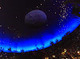# Plotting Points

### Overview

To plot a point, enter your values as (x,y):You can also add multiple points on a line by separating each coordinate with a comma:It's also possible to add points within a table:### More Resources

0 out of 2 found this helpful
Have more questions? Submit a request

•Chris Mackenzie
How to change points into a line?
•Team Desmos
Hi Chris, Try the regressions tour in the help menu (?) to get started creating curves of best fit. If you want to learn more, check out the Regressions page at http://learn.desmos.com/regressions. Happy graphing!
•Max Larose

How do I get every positive integer to show up as a points instead of lines? For example, instead of having a curve for y = x^2, just the points where x is a ehole number.

•Marc Dion

I'm trying to plot the focal points of an ellipse by first using the formula c^2=a^2-b^2 and then using the variable 'c' in point plotting form however this fails with a warning of "Too many variables".  Is this not possible to do?  I've sort of gotten around this by plotting the ellipse formula using 'x' instead of 'c' but this gives vertical lines at the focal points so I'd still prefer to find a way to plot the points dynamically as 'a' and 'b' change.•Antonio Raphael Walker

3,0

•Naelson17

How to sum two points?

When I try:
(1,2) + (2,3)

It throws me an error

•Royalbeatle

Marc Dion, I have the same problem. If someone finds an answer, please post it! I'm struggling.

•Fasteroid

@Naelson17,  the better question to ask is how to go about reading the x and y components of points.  If you can do that, then you can sum those components as described.

I myself had been wanting to be able to read the x and y components of points for various reasons, but really had no clue how to do so.  The epiphany came when I realized I could triangulate the position of the original point using three other points.  That's exactly what this graph does:You should be able to do just about any kind of point arithmetic your heart desires now.  Just rip the x and y out of your original points before doing anything else.

Cheers, and happy calculating.

Edited by Fasteroid
•Naelson17

@Fasteroid
I really liked your solution. I'll for sure use that a lot on my graphs.

Thanks for sharing the idea.

•Maverick Reynolds

Thank you so much for your solution Fasteroid. Implementing this will surely make my graph's "code" less clunky.

If I didn't find this, I would have defined points as a list and accessed each item in the list, which is okay I guess, but this is splendid